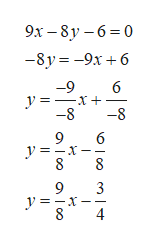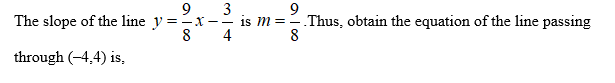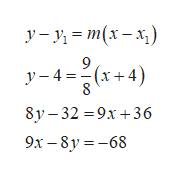# Find an equation of the line that passes through the point(−4, 4)and is parallel to the line9x − 8y − 6 = 0.(Let x be the independent variable and y be the dependent variable.)

Question
15 views

Find an equation of the line that passes through the point

(−4, 4)

and is parallel to the line

9x − 8y − 6 = 0.

(Let x be the independent variable and y be the dependent variable.)

check_circle

Step 1

Rewrite the equation 9x–8y–6=0 as,help_outlineImage Transcriptionclose9.х — 8у -6-0 -8y-9x6 -9 6 y =_ -8 -8 9 6 y=_ 8 8 9 3 8 4 fullscreen
Step 2...help_outlineImage TranscriptioncloseУ - у 3 т(х— х,) у —4 3D- (х +4) 8 8у-32 39х +36 9х — 8у %3 -68 fullscreen

### Want to see the full answer?

See Solution

#### Want to see this answer and more?

Solutions are written by subject experts who are available 24/7. Questions are typically answered within 1 hour.*

See Solution
*Response times may vary by subject and question.
Tagged in

### Other